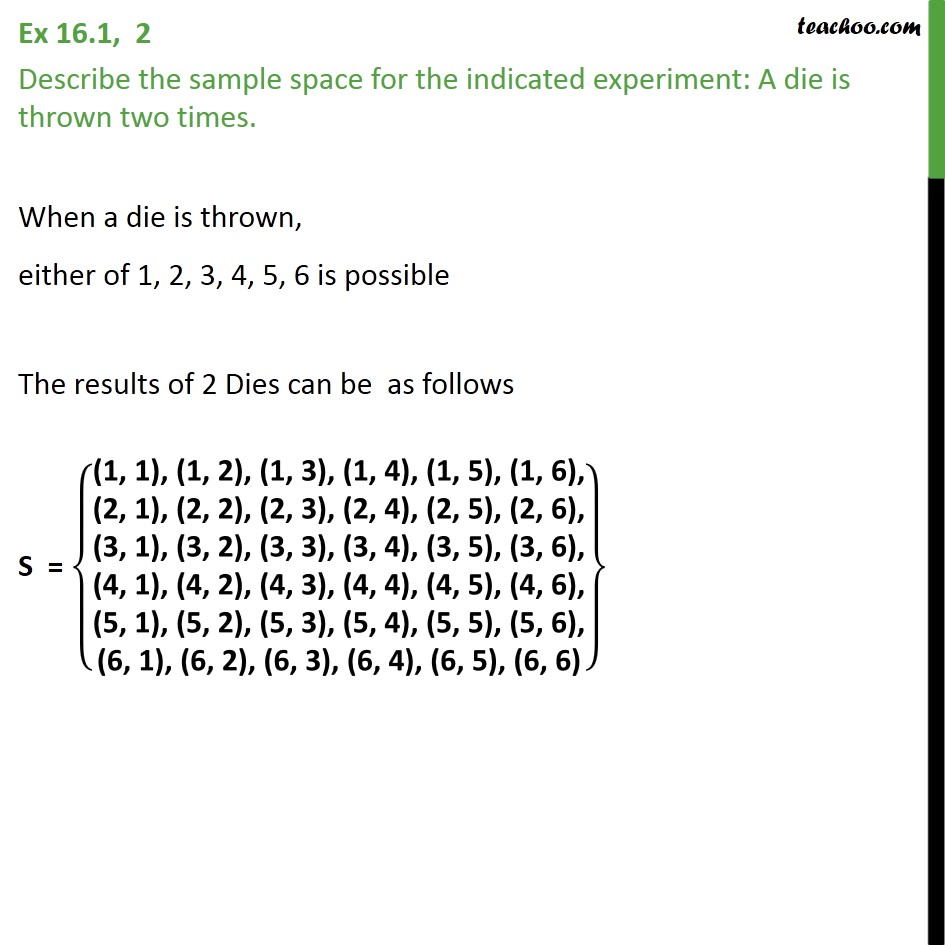Sample Space

Chapter 14 Class 11 Probability
Serial order wiseLearn in your speed, with individual attention - Teachoo Maths 1-on-1 Class

### Transcript

Question 2 Describe the sample space for the indicated experiment: A die is thrown two times. When a die is thrown, either of 1, 2, 3, 4, 5, 6 is possible The results of 2 Dies can be as follows S = (1, 1), (1, 2), (1, 3), (1, 4), (1, 5), (1, 6),﷮(2, 1), (2, 2), (2, 3), (2, 4), (2, 5), (2, 6),﷮(3, 1), (3, 2), (3, 3), (3, 4), (3, 5), (3, 6),﷮(4, 1), (4, 2), (4, 3), (4, 4), (4, 5), (4, 6),﷮(5, 1), (5, 2), (5, 3), (5, 4), (5, 5), (5, 6),﷮(6, 1), (6, 2), (6, 3), (6, 4), (6, 5), (6, 6)﷯﷯